# Nuclear Reactions QuestionsPage 1

#### WATCH ALL SLIDES

Slide 1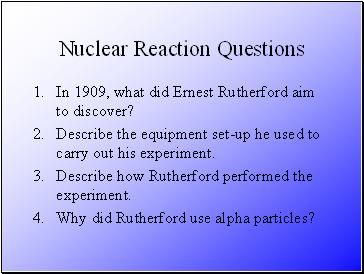## Nuclear Reaction Questions

In 1909, what did Ernest Rutherford aim to discover?

Describe the equipment set-up he used to carry out his experiment.

Describe how Rutherford performed the experiment.

Why did Rutherford use alpha particles?

Slide 2Nuclear Reaction Questions

As a result of his experiment, what 3 things did he observe?

What 3 conclusions did Rutherford make as a result of his findings?

Describe Rutherford’s model of the atom?

Slide 3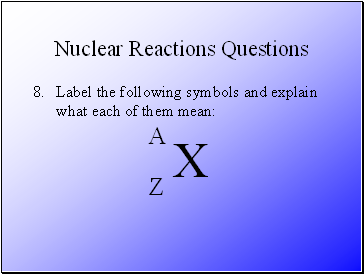Nuclear Reactions Questions

Label the following symbols and explain what each of them mean:

Slide 4Nuclear Reactions Questions

Describe how you would find the number of neutrons that an element contains?

Explain what an isotope is?

Slide 5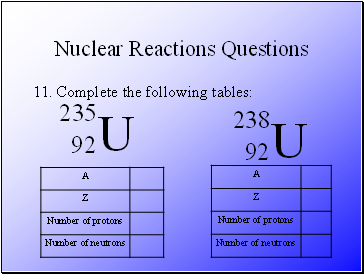Nuclear Reactions Questions

Complete the following tables:

Slide 6Nuclear Reactions Questions

What one thing is always produced as a result of radioactive decay?

What are the 3 types of radioactive decay that can occur?

What is an alpha particles equivalent to?

What is the equation rule for alpha decay?

Slide 7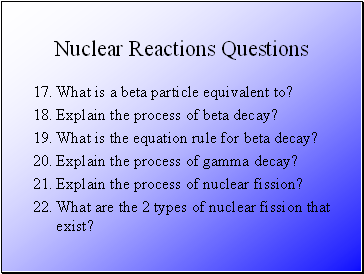Nuclear Reactions Questions

What is a beta particle equivalent to?

Explain the process of beta decay?

What is the equation rule for beta decay?

Explain the process of gamma decay?

Explain the process of nuclear fission?

What are the 2 types of nuclear fission that exist?

Slide 8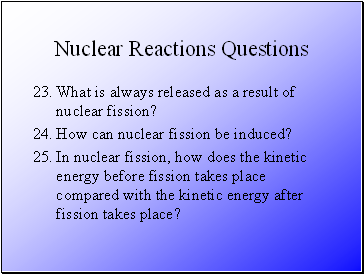Nuclear Reactions Questions

What is always released as a result of nuclear fission?

How can nuclear fission be induced?

In nuclear fission, how does the kinetic energy before fission takes place compared with the kinetic energy after fission takes place?

Slide 9Nuclear Reactions Questions

Explain the process of nuclear fusion?

What is always released as a result of nuclear fusion?

What is the equation which is used to calculate the amount of energy released during fission and fusion?

Slide 10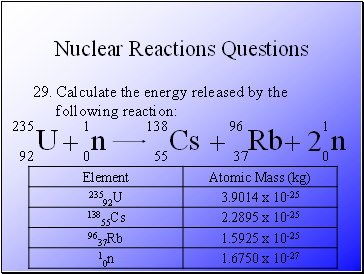Nuclear Reactions Questions

Calculate the energy released by the following reaction:

Go to page:
1  2